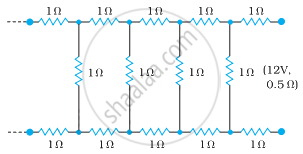# Determine the current drawn from a 12 V supply with internal resistance 0.5 Ω by the infinite network shown in the figure. Each resistor has 1 Ω resistance. - Physics

Numerical

Determine the current drawn from a 12 V supply with internal resistance 0.5 Ω by the infinite network shown in the figure. Each resistor has 1 Ω resistance.#### Solution

The resistance of each resistor connected in the given circuit, R = 1 Ω

Equivalent resistance of the given circuit = R’

The network is infinite. Hence, equivalent resistance is given by the relation,

∴ R' = 2 + "R'"/(("R'" + 1))

("R'")^2 - 2"R'" - 2 = 0

R' = (2 ± sqrt(4 + 8))/2

= (2 ± sqrt12)/2

= 1 ± sqrt3

Negative value of R’ cannot be accepted. Hence, equivalent resistance,

R' = (1 + sqrt3) = 1 + 1.73 = 2.73 Ω

Internal resistance of the circuit, r = 0.5 Ω

Hence, total resistance of the given circuit = 2.73 + 0.5 = 3.23 Ω

Supply voltage, V = 12 V

According to Ohm’s Law, current drawn from the source is given by the ratio, 12/3.23 = 3.72 A

Concept: Kirchhoff’s Rules
Is there an error in this question or solution?

#### APPEARS IN

NCERT Physics Part 1 and 2 Class 12
Chapter 3 Current Electricity
Exercise | Q 3.21 | Page 130
NCERT Class 12 Physics Textbook
Chapter 3 Current Electricity
Exercise | Q 21 | Page 130

Share